# Continuum hypothesis

(diff) ← Older revision | Latest revision (diff) | Newer revision → (diff)

The hypothesis, due to G. Cantor (1878), stating that every infinite subset of the continuumis either equivalent to the set of natural numbers or toitself. An equivalent formulation (in the presence of the axiom of choice) is: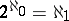(see Aleph). The generalization of this equality to arbitrary cardinal numbers is called the generalized continuum hypothesis (GCH): For every ordinal number,(1)

In the absence of the axiom of choice, the generalized continuum hypothesis is stated in the form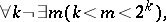(2)

where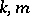stand for infinite cardinal numbers. The axiom of choice and (1) follow from (2), while (1) and the axiom of choice together imply (2).

D. Hilbert posed, in his celebrated list of problems, as Problem 1 that of proving Cantor's continuum hypothesis (the problem of the continuum). This problem did not yield a solution within the framework of traditional set-theoretical methods of solution. Among mathematicians the conviction grew that the problem of the continuum was in principle unsolvable. It was only after a way had been found of reducing mathematical concepts to set-theoretical ones, axioms had been stated in set-theoretical language which could be placed at the foundations of mathematical proofs actually encountered in real life and logical derivation methods had been formalized, that it became possible to give a precise statement, and then to solve the question, of the formal unsolvability of the continuum hypothesis. Formal unsolvability is understood in the sense that there does not exist a formal derivation in the Zermelo–Fraenkel system ZF either for the continuum hypothesis or for its negation.

In 1939 K. Gödel established the unprovability of the negation of the generalized continuum hypothesis (and hence the unprovability of the negation of the continuum hypothesis) in the system ZF with the axiom of choice (the system ZFC) under the hypothesis that ZF is consistent (see Gödel constructive set). In 1963 P. Cohen showed that the continuum hypothesis (and therefore also the generalized continuum hypothesis) cannot be deduced from the axioms of ZFC assuming the consistency of ZF (see Forcing method).

Are these results concerning the problem of the continuum final? The answer to this question depends on one's relation to the premise concerning the consistency of ZF and, what is more significant, to the experimental fact that every meaningful mathematical proof (of traditional classical mathematics) can, after it has been found, be adequately stated in the system ZFC. This fact cannot be proved nor can it even be precisely stated, since each revision raises a similar question concerning the adequacy of the revision for the revised theorem.

In model-theoretic language, Gödel and Cohen constructed models for ZFC in whichwhereis an arbitrary uncountable regular cardinal number given in advance, andis the first cardinal number greater than. What is the possible behaviour of the function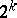in various models of ZFC?

It is known that for regular cardinal numbers, this function can take them to arbitrary cardinal numbers subject only to the conditions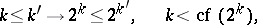where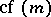is the smallest cardinal number cofinal with(see Cardinal number). For singular (that is, non-regular), the value of the functionmay depend on its behaviour at smaller cardinal numbers. E.g., if (1) holds for all, then it also holds for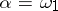.

How to Cite This Entry:
Continuum hypothesis. Encyclopedia of Mathematics. URL: http://encyclopediaofmath.org/index.php?title=Continuum_hypothesis&oldid=11759
This article was adapted from an original article by V.N. Grishin (originator), which appeared in Encyclopedia of Mathematics - ISBN 1402006098. See original article